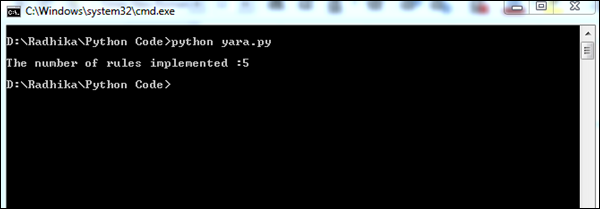# Python Forensics - Memory and Forensics

In this chapter, we will focus on investigating the volatile memory with the help of Volatility, a Python-based forensics framework applicable on the following platforms: Android and Linux.

## Volatile Memory

Volatile memory is a type of storage where the contents get erased when the system's power is turned off or interrupted. RAM is the best example of a volatile memory. It means, if you were working on a document that has not been saved to a non-volatile memory, such as a hard drive, and the computer lost power, then all the data will be lost.

In general, volatile memory forensics follow the same pattern as other forensic investigations −

• Selecting the target of the investigation
• Acquiring forensic data
• Forensic analysis

The basic volatility plugins which are used for Android gathers RAM dump for analysis. Once the RAM dump is gathered for analysis, it is important to start hunting for malware in RAM.

## YARA Rules

YARA is a popular tool which provides a robust language, is compatible with Perl-based Regular Expressions, and is used to examine the suspected files/directories and match strings.

In this section, we will use YARA based on the pattern matching implementation and combine them with utility power. The complete process will be beneficial for forensic analysis.

### Example

Consider the following code. This code helps in extracting the code.

```import operator
import os
import sys

sys.path.insert(0, os.getcwd())
import plyara.interp as interp

# Plyara is a script that lexes and parses a file consisting of one more Yara
# rules into a python dictionary representation.
if __name__ == '__main__':
file_to_analyze = sys.argv
authors = {}
imps = {}
meta_keys = {}
max_strings = []
max_string_len = 0
tags = {}
rule_count = 0

for rule in rulesDict:
rule_count += 1

# Imports
if 'imports' in rule:
for imp in rule['imports']:
imp = imp.replace('"','')

if imp in imps:
imps[imp] += 1
else:
imps[imp] = 1
# Tags
if 'tags' in rule:
for tag in rule['tags']:
if tag in tags:
tags[tag] += 1
else:
tags[tag] = 1

if key in meta_keys:
meta_keys[key] += 1
else:
meta_keys[key] = 1

if key in ['Author', 'author']:
else:

#Strings
if 'strings' in rule:
for strr in rule['strings']:
if len(strr['value']) > max_string_len:
max_string_len = len(strr['value'])
max_strings = [(rule['rule_name'], strr['name'], strr['value'])]
elif len(strr['value']) == max_string_len:
max_strings.append((rule['rule_name'], strr['key'], strr['value']))

print("\nThe number of rules implemented" + str(rule_count))
ordered_meta_keys = sorted(meta_keys.items(), key = operator.itemgetter(1),
reverse = True)
ordered_authors = sorted(authors.items(), key = operator.itemgetter(1),
reverse = True)
ordered_imps = sorted(imps.items(), key = operator.itemgetter(1), reverse = True)
ordered_tags = sorted(tags.items(), key = operator.itemgetter(1), reverse = True)
```

The above code will produce the following output.The number of YARA rules implemented helps in giving a better picture of the suspected files. Indirectly, the list of suspected files help in gathering appropriate information for forensics.

Following is the source code in github: https://github.com/radhikascs/Python_yara